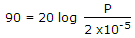# Electronics and Communication Engineering - Measurements and Instrumentation

### Exercise :: Measurements and Instrumentation - Section 1

6.

If reference sound pressure P0 is 2 x 10-5 N/m2, a sound pressure of 90 dB is equal to

 A. 0.632 N/m2 B. 0.707 N/m2 C. 0.835 N/m2 D. 0.925 N/m2

Explanation:.

7.

Which of the following voltmeters would you use for measuring voltage across 20 kΩ resistance?

 A. Voltmeter having a resistance of 5 kΩ B. Voltmeter having a sensitivity of 1 kW/V C. Voltmeter having sensitivity of 10 kW/V D. None of the above

Explanation:

Resistance of voltmeter should be very high as compared to 20 kΩ.

8.

A single phase energy meter has the rating 1200 resolutions/ kWh. If a 500 W electric gadget is used for 4 hours, the energy meter will make

 A. 1200 revolutions B. 1800 revolutions C. 2100 revolutions D. 2400 revolutions

Explanation:

0.5 x 4 = 2 kWh = 2 x 1200 revolutions.

9.

Assertion (A): De sauty's bridge is suitable only for pure capacitor.

Reason (R): Capacitors are mostly perfect.

 A. Both A and R are true and R is correct explanation of A B. Both A and R are true but R is not correct explanation of A C. A is true R is false D. A is false R is true

Explanation:

Capacitors are mostly imperfect.

10.

In which of the transformer is the secondary nearly short circuited under normal operating conditions?

 A. CT B. PT C. Distribution transformer D. Power transformer